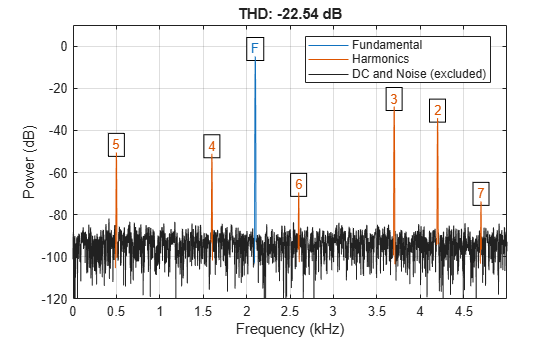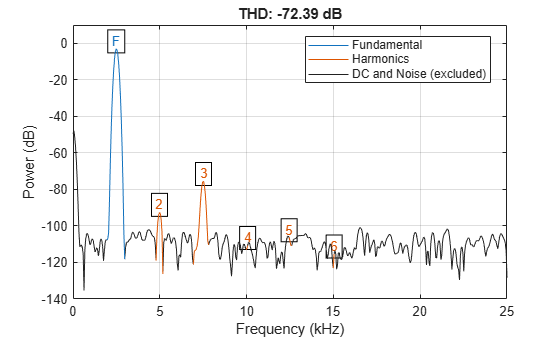# thd

Total harmonic distortion

## Syntax

``r = thd(x)``
``r = thd(x,fs,n)``
``r = thd(pxx,f,"psd")``
``r = thd(pxx,f,n,"psd")``
``r = thd(sxx,f,rbw,"power")``
``r = thd(sxx,f,rbw,n,"power")``
``r = thd(___,"aliased")``
``````[r,harmpow,harmfreq] = thd(___)``````
``thd(___)``

## Description

example

````r = thd(x)` returns the total harmonic distortion (THD) in dBc of the real-valued sinusoidal signal `x`. The total harmonic distortion is determined from the fundamental frequency and the first five harmonics using a modified periodogram of the same length as the input signal. The modified periodogram uses a Kaiser window with β = 38.```

example

````r = thd(x,fs,n)` specifies the sample rate `fs` and the number of harmonics (including the fundamental) to use in the THD calculation.```
````r = thd(pxx,f,"psd")` specifies the input `pxx` as a one-sided power spectral density (PSD) estimate. `f` is a vector of frequencies corresponding to the PSD estimates in `pxx`.```

example

````r = thd(pxx,f,n,"psd")` specifies the number of harmonics (including the fundamental) to use in the THD calculation.```

example

````r = thd(sxx,f,rbw,"power")` specifies the input as a one-sided power spectrum. `rbw` is the resolution bandwidth over which each power estimate is integrated.```
````r = thd(sxx,f,rbw,n,"power")` specifies the number of harmonics (including the fundamental) to use in the THD calculation.```

example

````r = thd(___,"aliased")` reports harmonics of the fundamental that are aliased into the Nyquist range. Use this option when the input signal is undersampled. If you do not specify this option, or if you set it to `"omitaliases"`, then the function ignores any harmonics of the fundamental frequency that lie beyond the Nyquist range.```

example

``````[r,harmpow,harmfreq] = thd(___)``` returns the powers (in dB) and frequencies of the harmonics, including the fundamental.```

example

````thd(___)` with no output arguments plots the spectrum of the signal and annotates the harmonics in the current figure window. It uses different colors to draw the fundamental component, the harmonics, and the DC level and noise. The THD appears above the plot. The fundamental and harmonics are labeled. The DC term is excluded from the measurement and is not labeled.```

## Examples

collapse all

This example shows explicitly how to calculate the total harmonic distortion in dBc for a signal consisting of the fundamental and two harmonics. The explicit calculation is checked against the result returned by `thd`.

Create a signal sampled at 1 kHz. The signal consists of a 100 Hz fundamental with amplitude 2 and two harmonics at 200 and 300 Hz with amplitudes 0.01 and 0.005. Obtain the total harmonic distortion explicitly and using `thd`.

```t = 0:0.001:1-0.001; x = 2*cos(2*pi*100*t)+0.01*cos(2*pi*200*t)+0.005*cos(2*pi*300*t); tharmdist = 10*log10((0.01^2+0.005^2)/2^2)```
```tharmdist = -45.0515 ```
`r = thd(x)`
```r = -45.0515 ```

Create a signal sampled at 1 kHz. The signal consists of a 100 Hz fundamental with amplitude 2 and three harmonics at 200, 300, and 400 Hz with amplitudes 0.01, 0.005, and 0.0025.

Set the number of harmonics to 3. This includes the fundamental. Accordingly, the power at 100, 200, and 300 Hz is used in the THD calculation.

```t = 0:0.001:1-0.001; x = 2*cos(2*pi*100*t)+0.01*cos(2*pi*200*t)+ ... 0.005*cos(2*pi*300*t)+0.0025*sin(2*pi*400*t); r = thd(x,1000,3)```
```r = -45.0515 ```

Specifying the number of harmonics equal to 3 ignores the power at 400 Hz in the THD calculation.

Create a signal sampled at 1 kHz. The signal consists of a 100 Hz fundamental with amplitude 2 and three harmonics at 200, 300, and 400 Hz with amplitudes 0.01, 0.005, and 0.0025.

Obtain the periodogram PSD estimate of the signal and use the PSD estimate as the input to thd. Set the number of harmonics to 3. This includes the fundamental. Accordingly, the power at 100, 200, and 300 Hz is used in the THD calculation.

```t = 0:0.001:1-0.001; fs = 1000; x = 2*cos(2*pi*100*t)+0.01*cos(2*pi*200*t)+ ... 0.005*cos(2*pi*300*t)+0.0025*sin(2*pi*400*t); [pxx,f] = periodogram(x,rectwin(length(x)),length(x),fs); r = thd(pxx,f,3,'psd')```
```r = -45.0515 ```

Determine the THD by inputting the power spectrum obtained with a Hamming window and the resolution bandwidth of the window.

Create a signal sampled at 10 kHz. The signal consists of a 100 Hz fundamental with amplitude 2 and three odd-numbered harmonics at 300, 500, and 700 Hz with amplitudes 0.01, 0.005, and 0.0025. Specify the number of harmonics to 7. Determine the THD.

```fs = 10000; t = 0:1/fs:1-1/fs; x = 2*cos(2*pi*100*t)+0.01*cos(2*pi*300*t)+ ... 0.005*cos(2*pi*500*t)+0.0025*sin(2*pi*700*t); [sxx,f] = periodogram(x,hamming(length(x)),length(x),fs,'power'); rbw = enbw(hamming(length(x)),fs); r = thd(sxx,f,rbw,7,'power')```
```r = -44.8396 ```

Generate a signal that resembles the output of a weakly nonlinear amplifier with a 2.1 kHz tone as input. The signal is sampled for 1 second at 10 kHz. Compute and plot the power spectrum of the signal. Use a Kaiser window with β = 38 for the computation.

```Fs = 10000; f = 2100; t = 0:1/Fs:1; x = tanh(sin(2*pi*f*t)+0.1) + 0.001*randn(1,length(t)); periodogram(x,kaiser(length(x),38),[],Fs,'power')```Harmonics stick out from the noise at frequencies of 4.2 kHz, 6.3 kHz, 8.4 kHz, 10.5 kHz, 12.6 kHz, and 14.7 kHz. All frequencies except for the first one are greater than the Nyquist frequency. The harmonics are aliased respectively into 3.7 kHz, 1.6 kHz, 0.5 kHz, 2.6 kHz, and 4.7 kHz.

Compute the total harmonic distortion of the signal. By default, `thd` treats the aliased harmonics as part of the noise.

`thd(x,Fs,7);`Repeat the computation, but now treat the aliased harmonics as part of the signal.

`thd(x,Fs,7,'aliased');`Create a signal sampled at 10 kHz. The signal consists of a 100 Hz fundamental with amplitude 2 and three odd-numbered harmonics at 300, 500, and 700 Hz with amplitudes 0.01, 0.005, and 0.0025. Specify the number of harmonics to 7. Determine the THD, the power at the harmonics, and the corresponding frequencies.

```fs = 10000; t = 0:1/fs:1-1/fs; x = 2*cos(2*pi*100*t)+0.01*cos(2*pi*300*t)+ ... 0.005*cos(2*pi*500*t)+0.0025*sin(2*pi*700*t); [r,harmpow,harmfreq] = thd(x,10000,7); [harmfreq harmpow]```
```ans = 7×2 100.0000 3.0103 201.0000 -321.0983 300.0000 -43.0103 399.0000 -281.9259 500.0000 -49.0309 599.0000 -282.1066 700.0000 -55.0515 ```

The powers at the even-numbered harmonics are on the order of $-300$ dB, which corresponds to an amplitude of $1{0}^{-15}$.

Generate a sinusoid of frequency 2.5 kHz sampled at 50 kHz. Add Gaussian white noise with standard deviation 0.00005 to the signal. Pass the result through a weakly nonlinear amplifier. Plot the THD.

```fs = 5e4; f0 = 2.5e3; N = 1024; t = (0:N-1)/fs; ct = cos(2*pi*f0*t); cd = ct + 0.00005*randn(size(ct)); amp = [1e-5 5e-6 -1e-3 6e-5 1 25e-3]; sgn = polyval(amp,cd); thd(sgn,fs);```The plot shows the spectrum used to compute the ratio and the region treated as noise. The DC level is excluded from the computation. The fundamental and harmonics are labeled.

## Input Arguments

collapse all

Real-valued sinusoidal input signal, specified as a row or column vector.

Example: `cos(pi/4*(0:159))+cos(pi/2*(0:159))`

Data Types: `single` | `double`

Sample rate, specified as a positive scalar. The sample rate is the number of samples per unit time. If the unit of time is seconds, then the sample rate has units of Hz.

Number of harmonics, specified as a positive integer.

One-sided PSD estimate, specified as a real-valued, nonnegative column vector.

The power spectral density must be expressed in linear units, not decibels. Use `db2pow` to convert decibel values to power values.

Example: `[pxx,f] = periodogram(cos(pi./[4;2]*(0:159))'+randn(160,2))` specifies the periodogram PSD estimate of a noisy two-channel sinusoid sampled at 2π Hz and the frequencies at which it is computed.

Data Types: `single` | `double`

Cyclical frequencies corresponding to the one-sided PSD estimate, `pxx`, specified as a row or column vector. The first element of `f` must be 0.

Data Types: `double` | `single`

Power spectrum, specified as a real-valued nonnegative row or column vector.

The power spectrum must be expressed in linear units, not decibels. Use `db2pow` to convert decibel values to power values.

Example: ```[sxx,w] = periodogram(cos(pi./[4;2]*(0:159))'+randn(160,2),'power')``` specifies the periodogram power spectrum estimate of a two-channel sinusoid embedded in white Gaussian noise and the normalized frequencies at which it is computed.

Resolution bandwidth, specified as a positive scalar. The resolution bandwidth is the product of the frequency resolution of the discrete Fourier transform and the equivalent noise bandwidth of the window.

## Output Arguments

collapse all

Total harmonic distortion in dBc, returned as a real-valued scalar.

Power of the harmonics, returned as a real-valued scalar or vector expressed in dB. Whether `harmpow` is a scalar or a vector depends on the number of harmonics you specify as the input argument `n`.

Frequencies of the harmonics, returned as a nonnegative scalar or vector. Whether `harmfreq` is a scalar or a vector depends on the number of harmonics you specify as the input argument `n`.

collapse all

### Distortion Measurement Functions

The functions `thd`, `sfdr`, `sinad`, and `snr` measure the response of a weakly nonlinear system stimulated by a sinusoid.

When given time-domain input, `thd` performs a periodogram using a Kaiser window with large sidelobe attenuation. To find the fundamental frequency, the algorithm searches the periodogram for the largest nonzero spectral component. It then computes the central moment of all adjacent bins that decrease monotonically away from the maximum. To be detectable, the fundamental should be at least in the second frequency bin. Higher harmonics are at integer multiples of the fundamental frequency. If a harmonic lies within the monotonically decreasing region in the neighborhood of another, its power is considered to belong to the larger harmonic. This larger harmonic may or may not be the fundamental.

`thd` fails if the fundamental is not the highest spectral component in the signal.

Ensure that the frequency components are far enough apart to accommodate for the sidelobe width of the Kaiser window. If this is not feasible, you can use the `"power"` flag and compute a periodogram with a different window.

## Version History

Introduced in R2013b

expand all